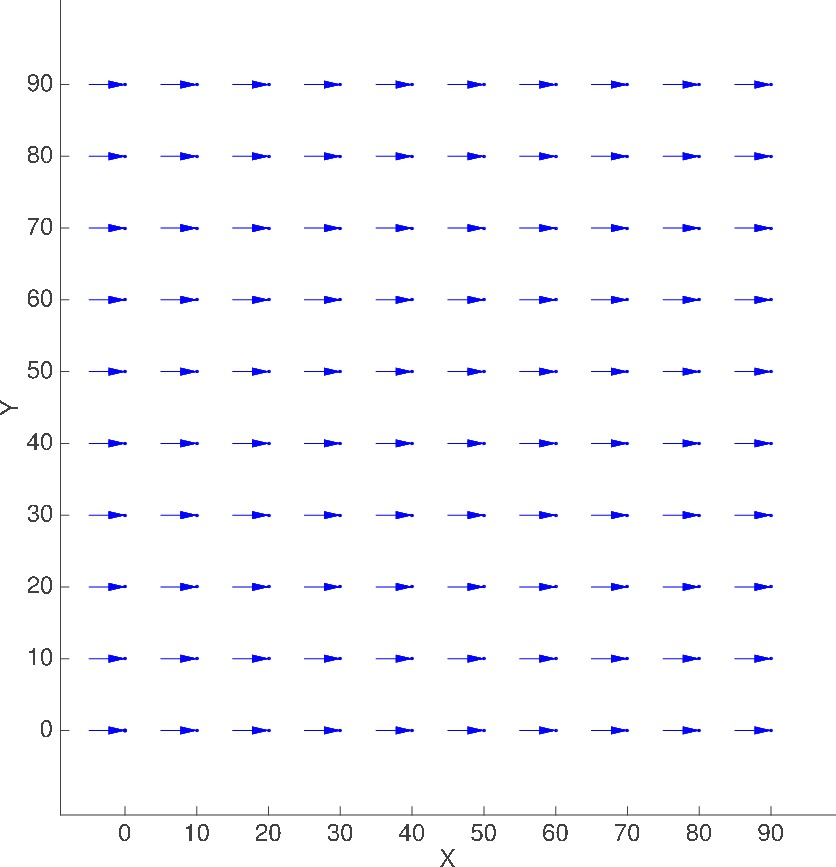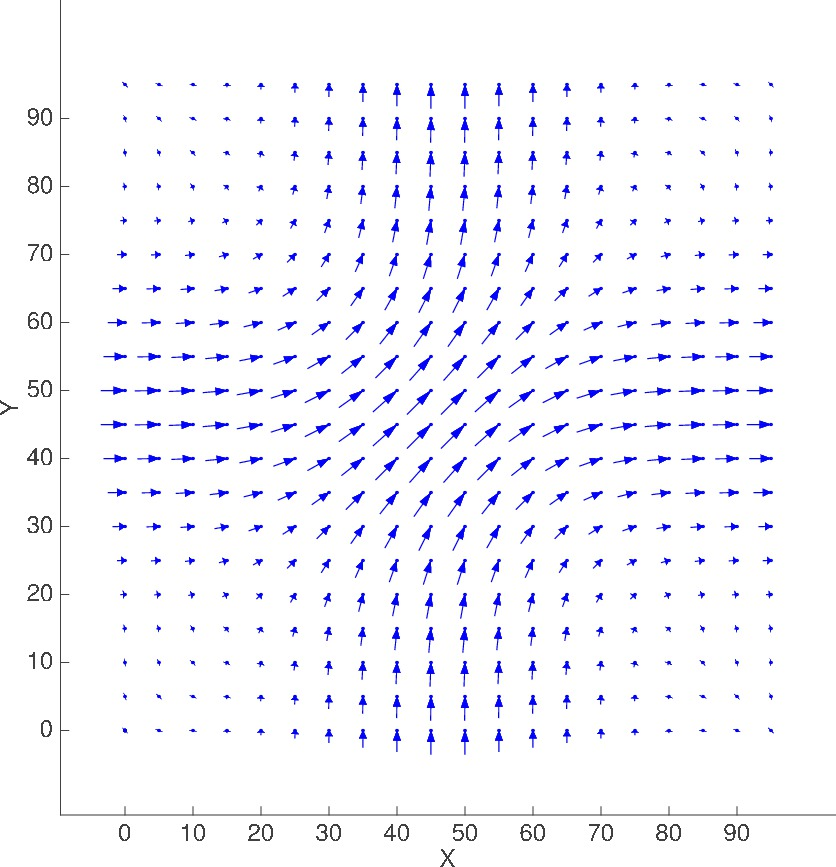Datasets for Causal Discovery

The data sets on this web site support the following paper:

Title: Using Causal Discovery to Track Information Flow in Spatio-Temporal Data - A Testbed and Experimental Results Using Advection-Diffusion Simulations
Authors: Imme Ebert-Uphoff, Yi Deng

Posted at arXiv.org: Dec 27, 2015.

Cite as:    arXiv:1512.08279 [cs.LG]

Description of data sets:
• Generated by: 2D Advection Diffusion Simulations
• Method: Numerical implementation of differential equations, namely the First-Order Upwind Scheme in two dimensions.

Motivation:

• To mimic the dominant physical processes in many geo science applications

• Advection is often described as a transport mechanism of a substance or property by a fluid (or air) due to the fluid's bulk motion.  In the context here we can think of an advection process as shifting a signal without changing its shape.
• The advection parameters are given by the advection velocity field which describes the speed and direction in which the signal is pushed.  It is usually scaled such that the highest velocity is about 1 m/sec.

Pure Diffusion:

• Diffusion causes a signal to spread while the center of the signal stays in place.  For example, a narrow wave of high amplitude is spread out into a wide wave with much lower amplitude.
• As diffusion parameter (kappa_x / kappa_y) we use 1 m*m/sec  (or 0 m*m/sec, if diffusion in any direction is turned off).

• The grid is rectangular, often consisting of 10x10 or 20x20 points.
• The simulation uses periodic boundary conditions, i.e. we use a wrap-around in both x- and y-direction.  For example, when reaching the right-most grid point in the x-direction, its neighbor to the right is the left-most grid point with the same y-coordinate.
• Parameter M defines whether we use full temporal resolution (M=1) from the simulations (Grid 1) also in the final data file (Grid 2) or reduce resolution by saving only every Mth sample in Grid 2.  This parameter is helpful to test algorithms for different signal speed and temporal resolutions.

Original purpose:
• To test algorithms for tracking information flow in Spatio-Temporal Data

Below you find:

1) Description of Scenarios
2) Description of Data files
3) Download - single tar directory containing all files for all scenarios.
4) A paper describing the simulation framework and the experiments we did with these data sets.

Created by:  Imme Ebert-Uphoff

1) Description of Scenarios

 Scenario Description (grid size, temporal resolution) M=1 --> every sample is saved M=2 --> every 2nd sample is saved M=4 --> every 4th sample is saved etc. Scenario name (XXX) Plot of Advection velocity field (displacement shown is in meters for t = 5 sec) A) SIMPLE SCENARIOS Pure Advection 10x10 grid, M=1 ADVECTION_ONLY_EXP_110x10, M=2 ADVECTION_ONLY_EXP_2 same as above 10x10, M=4 ADVECTION_ONLY_EXP_3 same as above 10x10, M=8 ADVECTION_ONLY_EXP_4 same as above 10x10, M=12 ADVECTION_ONLY_EXP_5 same as above 10x10, M=16 ADVECTION_ONLY_EXP_6 same as above 10x10, M=20 ADVECTION_ONLY_EXP_7 same as above 10x10, M=21 ADVECTION_ONLY_EXP_8 same as above 10x10, M=1, single-point noise ADVECTION_ONLY_INPUT_EFFECT_EXP_1 same as above 10x10, M=1, all-point noise ADVECTION_ONLY_INPUT_EFFECT_EXP_2 same as above Pure Diffusion 10x10, M=1 DIFFUSION_ONLY_EXP_1 No advection field 10x10, M=2 DIFFUSION_ONLY_EXP_2 No advection field 10x10, M=4 DIFFUSION_ONLY_EXP_3 No advection field 20x20, M=1 DIFFUSION_ONLY_EXP_4 No advection field 20x20, M=2 DIFFUSION_ONLY_EXP_5 No advection field 20x20, M=4 DIFFUSION_ONLY_EXP_6 No advection field 10x10, M=1, kx=1, ky=0 DIFFUSION_ONLY_EXP_7 No advection field 20x20, M=1, kx=1, ky=0 DIFFUSION_ONLY_EXP_8 No advection field 10x10, M=1, single-point noise DIFFUSION_ONLY_INPUT_EFFECT_EXP_1 No advection field 10x10, M=1, all-point noise DIFFUSION_ONLY_INPUT_EFFECT_EXP_2 No advection field Advection and Diffusion 10x10, M=1, Straight advection field ADV_AND_DIFF_STRAIGHTB) COMPLEX SCENARIOS (complex 2D advection fields) Scenario #1 Ring-shaped advection 20x20, M=1 ADV_AND_DIFF_CIRCULAR_30_65Ring-shaped advection 20x20, M=4 ADV_AND_DIFF_CIRCULAR_30_65_M_4 same as above Ring-shaped advection 20x20, M=10 ADV_AND_DIFF_CIRCULAR_30_65_M_10 same as above Scenario #2 Circular advection with opposing velocities at boundary 20x20, M=1 ADV_AND_DIFF_CIRCULAR_0_100Scenario #3 Cross current advection, ywo straight currents crossing each other 20x20, M= ADV_AND_DIFF_CROSS_CURRENT2) Description of Data Files

For each scenario there are several different data files, which are described in the table below.

 Type of file Parameter file Grid 1 coordinates Coordinates of advection field Advection field plot Grid 2 coordinates DATA FILE (time series data) FILE NAME, where XXX is the scenario name from the table above. XXX_PARAMETERS.m XXX_Grid1.txt XXX_ADVECTION_VEL.txt XXX_adv_vel_plot_5.tif XXX_Grid2.txt XXX_TIME_SERIES_DATA.txt DESCRIPTION Matlab file containing all input parameters that define the scenario.  While this is a Matlab file, it should be easy to understand even for people not familiar with Matlab. Time step and coordinates of grid points used for numerical simulations. Provided for simplicity.  This file is redundant, since the same coordinates are also included in XXX_ADVECTION_VEL.txt Advection velocity field used in the simulation.  Specifies the velocities at all grid points of Grid 1. (The file contains for each grid point the point coordinates, and the velocity at that point.  The coordinates always match those listed in XXX_Grid1.txt, but are included in this file, too, for convenience.) Plot of advection velocity field, showing displacement for t=5 sec. This is just provided for easy visualization of the scenario. Time step and coordinates of grid points corresponding to time series data file. (Only difference to Grid 1: resolution may be smaller in either time or space for Grid 2.) This is the actual data file, containing time series data for all grid points of Grid 2. SAMPLE FILES: Files for scenario XXX= ADV_AND_DIFF_CIRCULAR_30_65 XXX_PARAMETERS.m XXX_30_65_Grid_1.txt XXX_ADVECTION_VEL.txt XXX_adv_vel_plot_5.jpg XXX_Grid_2.txt XXX_TIME_SERIES_DATA.txt

Information for the interpretation of the TIME_SERIES_DATA file:
• The first line of the file contains all the variable names, called N1, N2, N3, ...   Each variable, N_i, contains the time series data for one grid point P_i.
• The number of grid points is determined by the scenario - typically either a 10x10 grid (thus variables N1 to N100) or a 20x20 grid (N1 to N400).
• The order of the grid points is identical to the one used in the Grid2 file.  Thus the X,Y-coordinates of each grid point can be read from file XXX_Grid2.txt.
• Each line (except for the first) contains one sample, i.e. one value for each grid point.
• The time series data actually consists of many separate runs that are concatenated.  There is one run generated for each grid point, so that each point gets the same set of initial conditions.
• If you want to separate the samples into individual runs, just take the total number of samples in the file and divide by the number of grid points, which will yield the number of samples for each run, say S.  The first S samples belong to the first run, the second set of S samples belongs to the second run, etc.

 Version Date Filename Comments 0 May 8, 2015 Sample files above Complete set of files for a single scenario, given in table above. You may want to just download those files first. 1.0 May 10, 2015 Combined tar-file (compressed tar file, 55 MB, expands to 400 MB !) First full version.  Contains all files for all scenarios listed above.

Please give me feedback on these files and the description.
By doing so you help me make these data sets useful to the community!

Contact:  Imme Ebert-Uphoff (iebert@engr.colostate.edu)

Last updated: Dec 30, 2015.